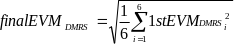## E.4 Derivation of the results

36.521-13GPPEvolved Universal Terrestrial Radio Access (E-UTRA)Part 1: Conformance testingRadio transmission and receptionRelease 17TSUser Equipment (UE) conformance specification

## E.4.1 EVM

For EVM create two sets of Z’(f,t)., according to the timing ”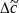–W/2 and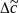+W/2” using the equalizer coefficients from E.3.3.

Perform the iDFTs on Z’(f,t). The IDFT-decoding preserves the meaning of t but transforms the variable f (representing the allocated sub carriers) into another variable g, covering the same count and representing the demodulated symbols. The samples in the post IDFT domain are called iZ’(g, t). The equivalent ideal samples are called iI(g,t). Those samples of Z’(f,t), carrying the reference symbols (=symbol 3) are not iDFT processed.

The EVM is the difference between the ideal waveform and the measured and equalized waveform for the allocated RB(s)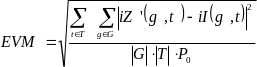,

where

t covers the count of demodulated symbols with the considered modulation scheme being active within the measurement period, (i.e. symbol 0,1,2,4,5and 6 in each slot, 🡪|T|=6 )

g covers the count of demodulated symbols with the considered modulation scheme being active within the allocated bandwidth. (|G|=12*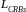(with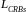: number of allocated resource blocks)).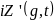are the samples of the signal evaluated for the EVM.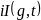is the ideal signal reconstructed by the measurement equipment, and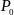is the average power of the ideal signal. For normalized modulation symbols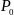is equal to 1.

From the acquired samples 40 EVM value can be derived, 20 values for the timing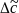–W/2 and 20 values for the timing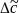+W/2

## E.4.2 Averaged EVM

EVM is averaged over all basic EVM measurements.

For subslot TTI, The averaging comprises 60 UL subslots (for frame structure 2: excluding special fields(UpPTS)) for PUCCH, PUSCH, PDSCH.

For subframe/slot TTI, the averaging comprises n UL slots (for frame structure 2: excluding special fields(UpPTS))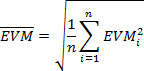where n is

n = 20 for PUCCH, PUSCH, PSDCH, PSCCH, and PSSCH,

n = 48 for PBSCH. The averaging is done separately for timing¦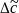–W/2 and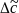+W/2 leading to and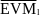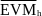is compared against the test requirements.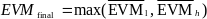## E.4.3 In-band emissions measurement

The in-band emissions are a measure of the interference falling into the non-allocated resources blocks.

Explanatory Note:

The inband emission measurement is only meaningful with allocated RBs next to non allocated RB. The allocated RBs are necessary but not under test. The non allocated RBs are under test. The RB allocation for this test is as follows: The allocated RBs are at one end of the channel BW, leaving the other end unallocated. The number of allocated RBs is smaller than half of the number of RBs, available in the channel BW. This means that the vicinity of the carrier in the centre is unallocated.

There are 3 types of inband emissions:

1. General

2. IQ image

3. Carrier leakage

Carrier leakage are inband emissions next to the carrier.

IQ image are inband emissions symmetrically (with respect to the carrier) on the other side of the allocated RBs.

General are applied to all unallocated RBs.

For each evaluated RB, the minimum requirement is calculated as the higher of PRB – 30 dB and the power sum of all limit values (General, IQ Image or Carrier leakage) that apply.

In specific the following combinations:

– Power (General)

– Power (General + Carrier leakage)

– Power (General + IQ Image)

1 and 2 is expressed in terms of power in one non allocated RB under test, normalized to the average power of an allocated RB (unit dB).

3 is expressed in terms of power in one non allocated RB, normalized to the power of all allocated RBs. (unit dBc).

This is the reason for two formulas Emissions relative.

Create one set of Y(t,f) per slot according to the timing “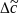For the non-allocated RBs below the in-band emissions are calculated as follows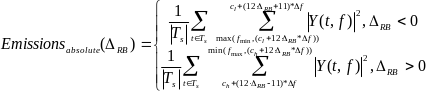,

where

the upper formula represents the in band emissions below the allocated frequency block and the lower one the in band emissions above the allocated frequency block.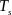is a set of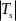SC-FDMA symbols with the considered modulation scheme being active within the measurement period,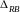is the starting frequency offset between the allocated RB and the measured non-allocated RB (e.g.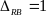for the first upper or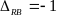for the first lower adjacent RB),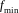and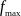are the lower and upper edge of the UL transmission BW configuration,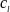and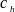are the lower and upper edge of the allocated BW,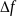is 15kHz,and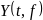is the frequency domain signal evaluated for in-band emissions as defined in the subsection E.3.3

The allocated RB power per RB and the total allocated RB power are given by: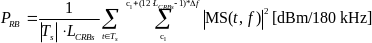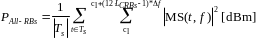The relative in-band emissions, applicable for General and IQ image, are given by: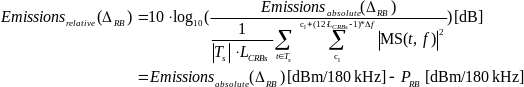where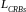is the number of allocated resource blocks,

and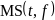is the frequency domain samples for the allocated bandwidth, as defined in the subsection E.3.3.

The relative in-band emissions, applicable for carrier leakage, is given by: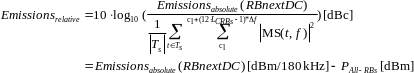where RBnextDC means: Resource Block next to the carrier.

This is one RB, namely the central one in case of an odd number of RBs in the channel BW.

This is one pair of RBs, namely the immediately adjacent RBs to the carrier in case of an even number of RBs in the channel BW.

Although an exclusion period may be applicable in the time domain, when evaluating EVM (clause E.7), the inband emissions measurement interval is defined over one complete slot in the time domain.

From the acquired samples 20 functions for general in band emissions and IQ image inband emissions can be derived. 20 values or 20 pairs of carrier leakage inband emissions can be derived. They are compared against different limits.

## E.4.4 EVM equalizer spectrum flatness

For EVM equalizer spectrum flatness use EC(f) as defined in E.3.3. Note, EC(f) represents equalizer coefficient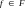，f is the allocated subcarriers within the transmission bandwidth ((|F|=12*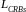)

From the acquired samples 20 functions EC(f) can be derived.

EC(f) is broken down to 2 functions: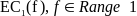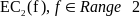Where Range 1 and Range 2 are as defined in Table 6.5.2.4.5-1 for normal condition and Table 6.5.2.4.5-2 for extreme condition

The following peak to peak ripple is calculated: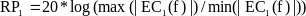,which denote the maximum ripple in Range 1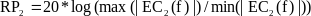,which denote the maximum ripple in Range 2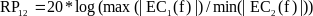,which denote the maximum ripple between the upper side of Range 1 and lower side of Range 2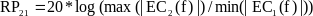,which denote the maximum ripple between the upper side of Range 2 and lower side of Range 1

See E.3.1.

## E.4.6 EVM of Demodulation reference symbols (EVMDMRS)

For the purpose of EVM DMRS, the steps E.2.2 to E.4.2 are repeated 6 times, constituting 6 EVM DMRS sub-periods. The only purpose of the repetition is to cover the longer gross measurement period of EVM DMRS (120 time slots) and to derive the FFT window timing per sub-period.

The bigger of the EVM results in one 20 TS period corresponding to the timing¦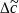–W/2 or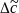+W/2 is compared against the limit. (Clause E.4.2) This timing is re-used for EVM DMRS in the equivalent EVM DMRS sub-period.

For EVM the demodulation reference symbols are excluded, while the data symbols are used. For EVMDMRS the data symbols are excluded, while the demodulation references symbols are used. This is illustrated in figure E.4.6-1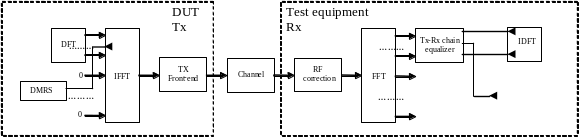Figure E.4.6-1: EVMDMRS measurement points

Re-use the following formula from E.3.3:

Z’(f,t) = MS(f,t) . EC(f)

To calculate EVMDMRS , the data symbol ( t=0,1,2,4,5,6) in Z’(f,t) are excluded and only the reference symbol (t=3) is used.

The EVM DMRS is the difference between the ideal waveform and the measured and equalized waveform for the allocated RB(s)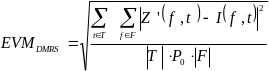,

where

t covers the count of demodulation reference symbols (i.e. only symbol 3 in each slot, so count =1)

f covers the count of demodulation reference symbols within the allocated bandwidth. (|F|=12*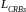(with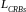: number of allocated resource blocks)).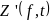are the samples of the signal evaluated for the EVM DMRS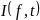is the ideal signal reconstructed by the measurement equipment, and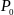is the average power of the ideal signal. For normalized modulation symbols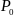is equal to 1.

20 such results are generated per measurement sub-period.

### E.4.6.1 1st average for EVM DMRS

EVM DMRS is averaged over all basic EVM DMRS measurements in one sub-period

For subslot TTI, The averaging comprises 60 UL subslots (for frame structure 2: excluding special fields(UpPTS)) for PUCCH, PUSCH, PDSCH.

For subframe/slot TTI, the averaging comprises 20 UL slots (for frame structure 2: excluding special fields(UpPTS))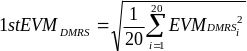The timing is taken from the EVM for the data. 6 of those results are achieved from the samples. In general the timing is not the same for each result.

### E.4.6.2 Final average for EVM DMRS